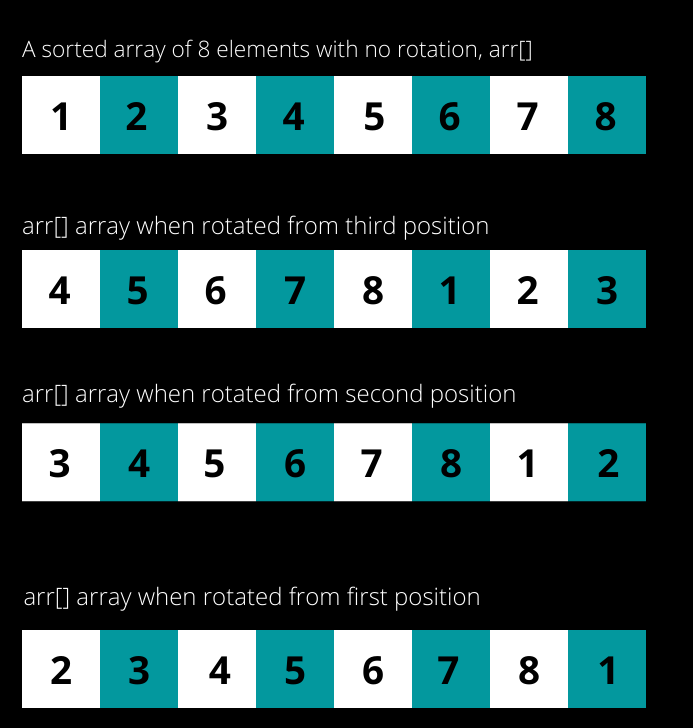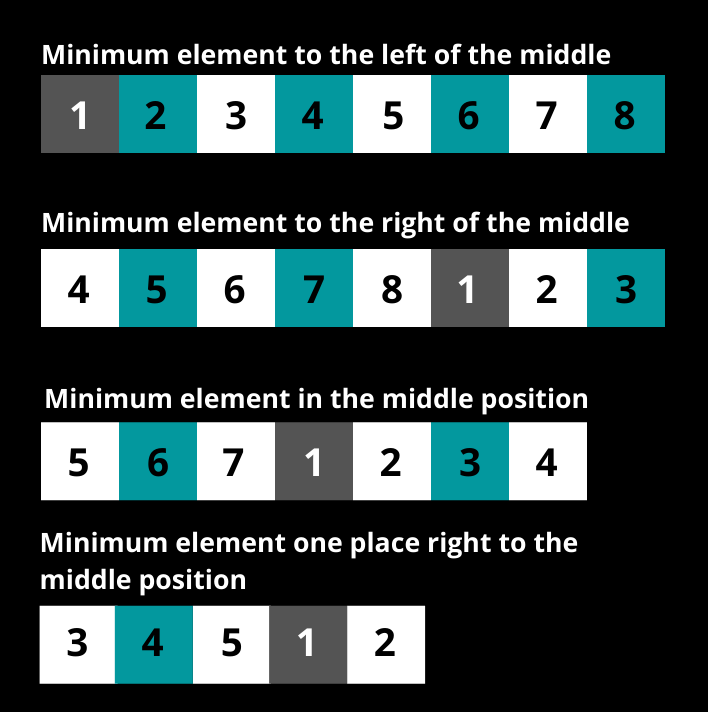# Find the minimum element in a sorted and Rotated Array

## Introduction

Searching your roll number in a set of lists is something we all have done at least once in our lives. The process is simple, linearly scan the list till you find your roll number.(Source: Amino Apps)

Searching and Sorting is one of the most basic operations for a computer program as well. Thousands of real-world applications like Twitter, Facebook, Instagram use the concept of searching one item from lakhs of items. For example, when you are searching a particular tweet from crores of tweets. Searching has to be done in a few milliseconds in such cases. The example was just for visualization purposes; the actual process of searching in such applications is quite a lot different.

Questions related to searching are frequently asked in technical interviews of companies in order to judge a candidate’s understanding of fundamental concepts of programming.

This blog will discuss an important interview problem: To find the minimum element in a sorted and rotated array.

## Finding the Minimum Element in a Sorted and Rotated Array: Problem Statement

The problem statement says, "You are given a sorted array of N integers. The array is rotated at some unknown point. Find the minimum element in the array."

### For Example:

Input: arr[] = {5, 6, 1, 3, 4}

Output: 1

Explanation: The minimum element in the array is 1

Input: arr[] = {4, 5, 6, 7, 0}

Output: 0

Explanation: The minimum element in the array is 0

## Approach 1: Finding the Minimum Element in a Sorted and Rotated Array

In an interview, it is always recommended to start with the brute force approach first and then try to optimize it.

The brute force approach for this question is to linearly scan the entire array of N elements and find the minimum element in the array. The time complexity for this approach is O(N), where N is the number of elements in the array.

public class LinearSearch {

public static int findMinimum(int[] arr)

{

int min = Integer.MAX_VALUE;

for(int i = 0; i < arr.length; i++)

{

if(arr[i] < min)

{

min = arr[i];

}

}

return min;

}

public static void main(String[] args) {

int[] arr1 = {6, 7, 8, 9, 10, 1, 2, 3, 4, 5};

System.out.println("The minimum element in the array is: " +findMinimum(arr1));

int[] arr2 = {0, 2, 3, 4, 5, 6, 7, 8, 9, 10};

System.out.println("The minimum element in the array is: " +findMinimum(arr2));

}

}

The output for the above program is:

The time complexity of the above program is O(N) where N is the size of the array and space complexity is O(1).

This approach does not utilize the fact that the array was initially sorted and then rotated at some point.

## Approach 2: Finding the Minimum Element in a Sorted and Rotated Array

Another possible approach to search an element is using Binary Search. However, binary search cannot be straightaway applied to this problem. As the sorted array is rotated at some point.

Before moving forward, Refer to the below image for an understanding of how array rotation works.A simple strategy to follow when you can’t figure out another way to solve a problem, examine the inputs and the possible outputs given. Let's try to take out another pattern using some examples.

On careful observation, you may observe that the minimum element is the only element whose previous is greater than it, as seen in examples 1, 2, and 3. If there is no previous element, then there is no rotation, and the first element is the minimum element, as seen in example 4.

So in a nutshell, by using binary search, the minimum element in a sorted and rotated array can be found in the following positions:

1. Minimum element to the left of the middle element.
2. Minimum element to the right of the middle element.
3. The minimum element in the middle position,
4. Minimum element in the (middle + 1) position.

The positions of the minimum element are summarized in the below image for all the above-mentioned conditions.### Algorithm

• Check if the middle element is the minimum element by comparing it with the element at position (mid - 1).
• Check if the element at the (mid+1) position is the minimum element by comparing it with the element at the mid position.
• If the minimum element is not at the mid and (mid + 1) position, then the minimum element lies in either the left or right half:
• If the middle element is smaller than the last element, then the minimum element lies in the left half.
• Else, the minimum element lies in the right half.

### Implementation

public class FindMinimum {

static int findMinimum(int[] arr, int left, int right) {

// Case 1: When the array is not rotated

// {1, 2, 3, 4}

if (right < left) {

return arr;

}

// Case 2: When the array contains only one element or after

// repeated iterations only one element is left to be compared

// {1}

if (right == left) {

return arr[left];

}

int mid = left + (right - left) / 2;

// Case 3: Check if the middle element is the minimum element

// by comparing it with the previous element.

// {5, 6, 7, 1, 2, 3, 4}

if (mid > left && arr[mid] < arr[mid - 1]) {

return arr[mid];

}

// Case 4: Check if the element at position ( mid + 1 ) is

// the minimum element by comparing it with the element at mid

// position

// {3, 4, 5, 1, 2}

if (mid < right && arr[mid + 1] < arr[mid]) {

return arr[mid + 1];

}

// Case 5: When the minimum element lies in the left half

// {1, 2, 3, 4, 5}

if (arr[right] > arr[mid]) {

return findMinimum(arr, left, mid - 1);

}

// Case 6: When the minimum element lies in the right half

// {3, 4, 5, 6, 7, 1, 2}

else {

return findMinimum(arr, mid + 1, right);

}

}

public static void main(String[] args) {

int[] arr1 = { 1, 2, 3, 4, 5, 6, 7, 8 };

System.out.println("Minimum element in arr1 is: ");

System.out.println(findMinimum(arr1, 0, arr1.length - 1));

int[] arr2 = { 4, 5, 6, 7, 8, 1, 2, 3 };

System.out.println("Minimum element in arr2 is: ");

System.out.println(findMinimum(arr2, 0, arr2.length - 1));

int[] arr3 = {3, 4, 5, 6, 7, 8, 1, 2};

System.out.println("Minimum element in arr2 is: ");

System.out.println(findMinimum(arr3, 0, arr3.length - 1));

int[] arr4 = {2, 3, 4, 5, 6, 7, 8, 1};

System.out.println("Minimum element in arr4 is: ");

System.out.println(findMinimum(arr4, 0, arr4.length - 1));

}

}

The output of the above program is:

The time complexity is O(log N), where N is the size of the array.

The space complexity is O(1), i.e., constant space complexity.

In the case of an array with all duplicate elements, the above approach will take O(N) time, i.e., worst-case complexity. To handle the case where an array may contain duplicate elements the Approach 3 is used.

## Approach 3: Finding the minimum element in a sorted and rotated array with duplicate elements

Approach 2 discussed above will take O(N) time in case of an array with duplicate elements.

To understand this, consider an array of 9 elements, {1, 1, 1, 1, 1, 1, 1, 1, 1} which is rotated by 1 position, so the array will become, {1, 1, 1, 1, 1, 1, 1, 1, 1}. Let’s quickly dry run this problem using the approach discussed above:

It can be easily concluded that even though the element at the first position was also one of the minimum elements, the approach discussed above still searches for the minimum element in the entire array making the overall time complexity to be O(N). Duplicate elements in an array need to be handled in better time complexity.

### Algorithm

• Initialize left and right with 0 and the size of the array respectively.
• Find the middle position of the array.
• Compare the elements in the order given below till the condition left < right is satisfied.
• Case 1: Check if the middle element is equivalent to the element at the right position. If this is true, then decrement the right.
• Case 2: If the middle element is greater than the element at the right position, then shift the left pointer by 1 position to the right of mid.
• Case 3: If none of the conditions holds true, then make the right pointer equivalent to the middle pointer.

The below program will handle duplicate elements to find the minimum element in a sorted and rotated array.

public class Demo {

public static int findMinimumOne(int[] arr, int left, int right) {

while (left < right) {

int mid = left + (right - left) / 2;

if (arr[mid] == arr[right]) {

right--;

} else if (arr[mid] > arr[right]) {

left = mid + 1;

} else {

right = mid;

}

}

return arr[right];

}

public static void main(String[] args) {

int[] arr1 = { 1, 2, 3, 4, 5, 6 };

System.out.println("The minimum element in the sorted and rotated array, arr1 is: " + findMinimumOne(arr1, 0, arr1.length - 1));

int[] arr2 = { 5, 6, 7, 1, 1, 2, 3, 4 };

System.out.println("The minimum element in the sorted and rotated array, arr2 is: " + findMinimumOne(arr2, 0, arr2.length - 1));

}

}

The output of the above program is:

1. How does Linear Search an array works?
Linear search works by linearly scanning/iterating over the entire array till the desired element is found.
For finding the minimum element in a sorted and rotated array, Linear Search can be used, as shown above.

2. Give examples of sorted and rotated arrays.
Consider a sorted array of 7 elements, say, {1, 2, 3, 4, 5, 6, 7}.
The array on rotation by 1 element will become, {2, 3, 4, 5, 6, 7, 1}.
The array on rotation by two elements will become, {3, 4, 5, 6, 7, 1, 2}.
The array on rotation by three elements will become, {4, 5, 6, 7, 1, 2, 3}.
The array on rotation by four elements will become, {5, 6, 7, 1, 2, 3, 4}.

## Key Takeaways

Arrays are important from an interview perspective, and almost all the major product-based companies ask questions about Arrays in the initial stages. Therefore it is important to have a good understanding of Arrays and practice a lot of good quality questions on them.

If you are preparing for an interview, you must refer to the Interview Guide for Product Based Companies guided path offered by Codestudio.
Remember, the more you practice, the more you grow. Practice is the key to success.